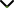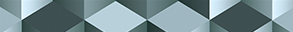# Fixed Point Theory and Applications for Function Spaces 2022

Publishing date
01 Jun 2023
Status
Closed
20 Jan 2023

Guest Editors

1King Abdulaziz University, Jeddah, Saudi Arabia

3Beijing Normal University, Beijing, China

This issue is now closed for submissions.

# Fixed Point Theory and Applications for Function Spaces 2022

This issue is now closed for submissions.

## DescriptionNumerous problems in science and engineering defined by nonlinear functional equations can be solved by reducing them to an equivalent fixed-point problem. In fact, an operator equation Gx = 0 may be expressed as a fixed-point equation Fx = x, where F is a self-mapping with some suitable domain. Fixed Point Theory provides essential tools for solving problems arising in various branches of mathematical analysis, such as split feasibility problems, variational inequality problems, nonlinear optimization problems, equilibrium problems, complementarity problems, selection and matching problems, and problems of proving the existence of solution of integral and differential equations.

Fixed point theorems are developed for single-valued or set-valued mappings of abstract metric spaces. In particular, the fixed-point theorems for set-valued mappings are rather advantageous in optimal control theory and have been frequently used to solve many problems in economics and game theory. On the other hand, in the case that F is non-self-mapping, the aforementioned equation does not necessarily have a fixed point. In such a case, it is worthy to determine an approximate solution x such that the error d(x, Tx) is minimum. This is the idea behind the best approximation theory.

The objective of this Special Issue is to report the latest advancements in the solutions of real-world problems, in particular by using the fixed/best-proximity point theory. The solvability of such problems is usually investigated in specific function spaces. The choice of the appropriate fixed/best-proximity point theorem and use the specific properties of the underlying function space can have a big impact on the solvability of nonlinear operator equations. We aim to provide a platform for researchers to promote, share, and discuss various new issues and developments in this area.

Potential topics include but are not limited to the following:

• Fixed point theory in various abstract spaces with applications
• Best proximity point theory in various abstract spaces with applications
• Existence of solutions of differential and integral equations via fixed point results
• Differential and integral inclusions by fixed point theory
• Stability of functional equations related to fixed point theory
• Fractional differential equations by fixed point theory
• Fractional differential inclusions by fixed point theoryJournal metrics
See full report
Acceptance rate23%
Submission to final decision72 days
Acceptance to publication20 days
CiteScore2.600
Journal Citation Indicator1.430
Impact Factor1.9Author guidelinesEditorial boardDatabases and indexing

Article of the Year Award: Impactful research contributions of 2022, as selected by our Chief Editors. Discover the winning articles.